### 15.2 Functions of the implementation

Table 15.1 lists the main functions of the explicit and implicit compressible flow solver. The discretization of the advection term mainly uses the ` AdvectVolumeFlux` and ` AdvectTraceFlux` to calculate the fluxes on the quadrature points of inside the element and on the element boundaries. Then, ` IProductWRTDerivBase` and ``` AddTraceIntegral``` are adopted to perform the integrations. Similarly, in the diffusion discretization, ` DiffuseVolumeFlux` and ` DiffuseTraceFlux` are implemented for calculating the fluxes, while ` IProductWRTDerivBase` and ` AddTraceIntegral` are for the integrations. For the diffusion term, spatial derivatives are needed and calculated using ``` DiffuseCalcDerivative```. For the interior penalty method an extra symmetric term may be needed and calculated by ` AddDiffusionSymmFluxToCoeff`. The above functions forms the spatial operator of ` DoOdeRhs` and the explicit solver in relatively complete coupled with a explicit time integration scheme. For the implicit solver, extra functions are needed. The ` DoImplicitSolve` solves the nonlinear system, in which the ` NonlinSysEvaluatorCoeff1D` evaluates the nonlinear system residual, ``` MatrixMultiplyMatrixFreeCoeff``` calculates the Jacobian matrix vector inner product and ``` PreconCoeff``` perform the preconditioning. Provided a specific implicit time integration scheme, the ` DoImplicitSolve` helps solve the nonlinear system for implicit stages and the implicit solver is complete.

Table 15.1 Table of variable and function mapping used in the compressible flow solver to their mathematical operations

 Variable/Function name Physical meaning ` AdvectVolumeFlux` Advection Euler flux: Fi ` AdvectTraceFlux` Advection (Riemann) numerical flux at trace: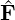i ` IProductWRTDerivBase` ∑ j=1dBT DjT Λ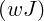operator to a vector ` AddTraceIntegral`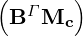T Λ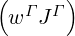to a vector ` MultiplyByElmImvMass` Multiply the inverse of mass matrix to a vector ` DiffuseCalcDerivative` Calculate the derivatives of a vector ` DiffuseVolumeFlux` Analytical Diffusion flux: G i ` DiffuseTraceFlux` Calculate the diffusion numerical flux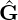` AddDiffusionSymmFluxToCoeff` Add the integral of symmetric flux (special for IP) ` DoOdeRhs` Calculate Lδ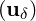` DoImplicitSolve` Solve the nonlinear system in implicit time integrations ` NonlinSysEvaluatorCoeff1D` Calculate N(uδ(i)) ` MatrixMultiplyMatrixFreeCoeff` Calculate ∂N∕∂u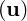⋅q ` PreconCoeff` Perform preconditioning